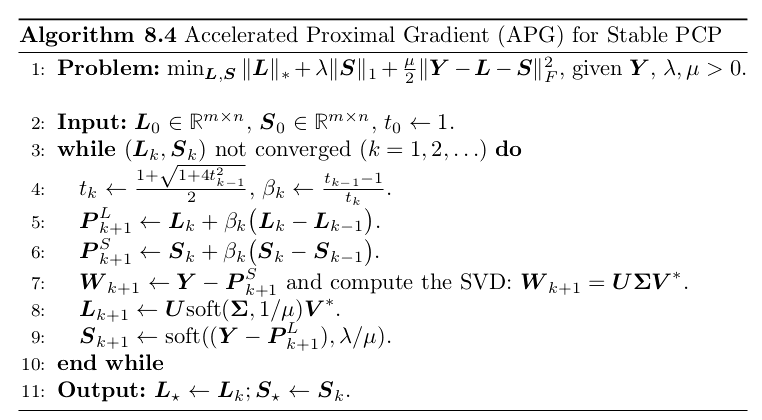### Lasso Regression

#### convergence rates of first order methods

$L>0$,那么这个$L$被称为Lipschitz常量。有了这个条件，我们可以给线性下界补充一个二次上界。

1. $g(x) = I_D$，指示函数，也就是对于一个凸集$D$,如果$x\in D$，$I_D(x) = 0$，否则$I_D(x) = \infty$。那么:
2. $g(\boldsymbol{x})=\lambda|\boldsymbol{x}|_{1}$，$l^1$范数：

3. $g(\boldsymbol{x})=\lambda|\boldsymbol{X}|_{*}$，矩阵核范数，那么：

$(\boldsymbol{U}, \boldsymbol{\Sigma}, \boldsymbol{V})$是$\mathbf W$的SVD分解。

$F(x) = f(x) + g(x)$，其中$f$是一个可导凸函数，并且导数符合Lipschitz，$g$是一个凸函数，考虑下面的迭代方式：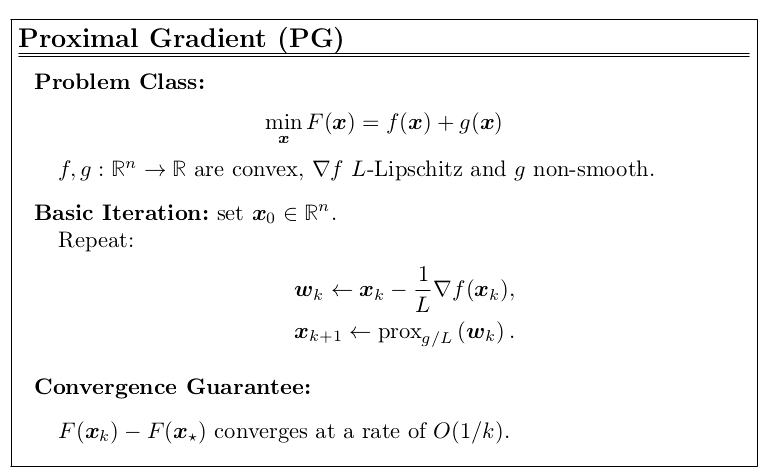1. Proximal Gradient for the Lasso
Lasso的$g$是$l^1$范数形式，而它的$L$可以是矩阵$A^TA$的最大特征值，s 可以提前计算得到的。有时候对于Lasso的PGD算法又被称为ISTA(iterative soft-thresholding algorithm)，具体算法流程如下图：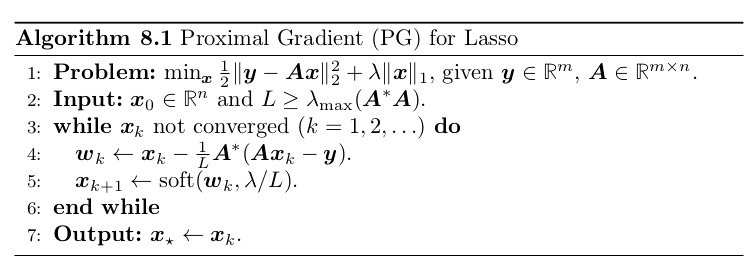2. Proximal Gradient for Stable PCP
观察之前不同形式的近端操作，可以看到对核范数$\Vert X\Vert_*$也是有y一个简单的近端形式的。因此我们可以用PGD来解决恢复低秩矩阵的问题，比如稳定主成分追踪（stable principal
component pursuit,PCP）:上式中有两个不可导项：各自都有比较简单的近端形式。我们还需要知道下面的内容，假设$\boldsymbol{x}=\left[\boldsymbol{x}_{1} ; \boldsymbol{x}_{2}\right] \text {, } g(\boldsymbol{x})=g_{1}\left(\boldsymbol{x}_{1}\right)+g_{2}\left(\boldsymbol{x}_{2}\right)$，那么:对于该问题的具体优化算法如下图：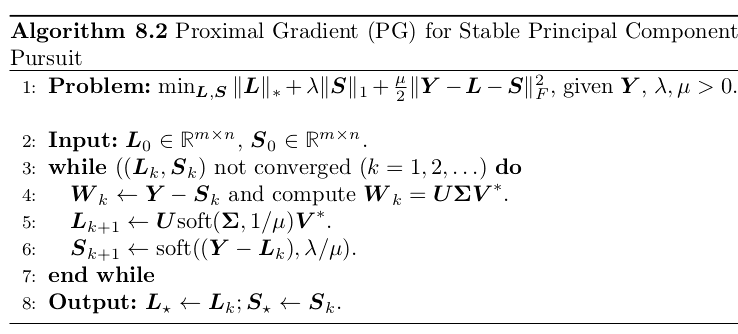1983年，Yuri Nesterov提出了达到收敛速度为$O(\frac{1}{k^2})$的算法。在算法中，他也使用到了动量这个步骤。它引入了一个新的辅助点（auxiliary point）$p_{k+1}$: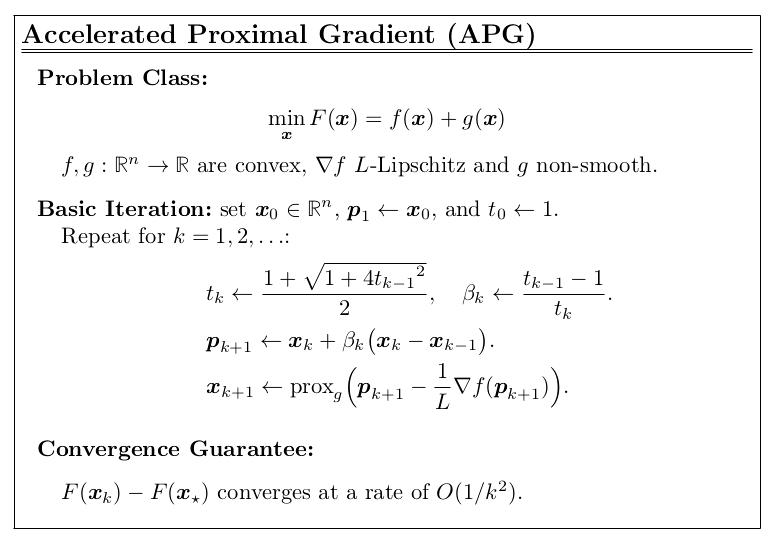APG算法有$O(\frac{1}{k^2})$的收敛速率，比普通的梯度方法会好很多，更正式的定理为：

1. APG for Basis Pursuit Denoising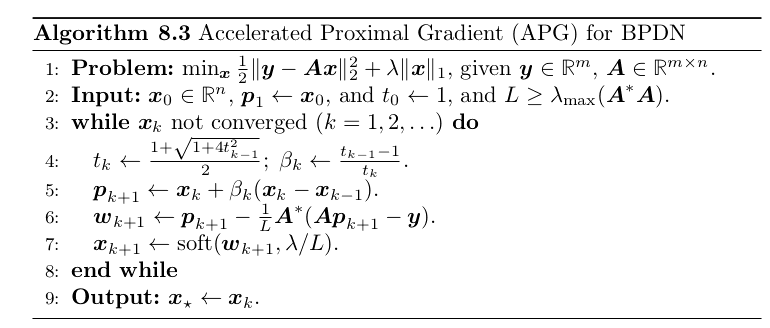2. APG for Stable Principal Component Pursuit